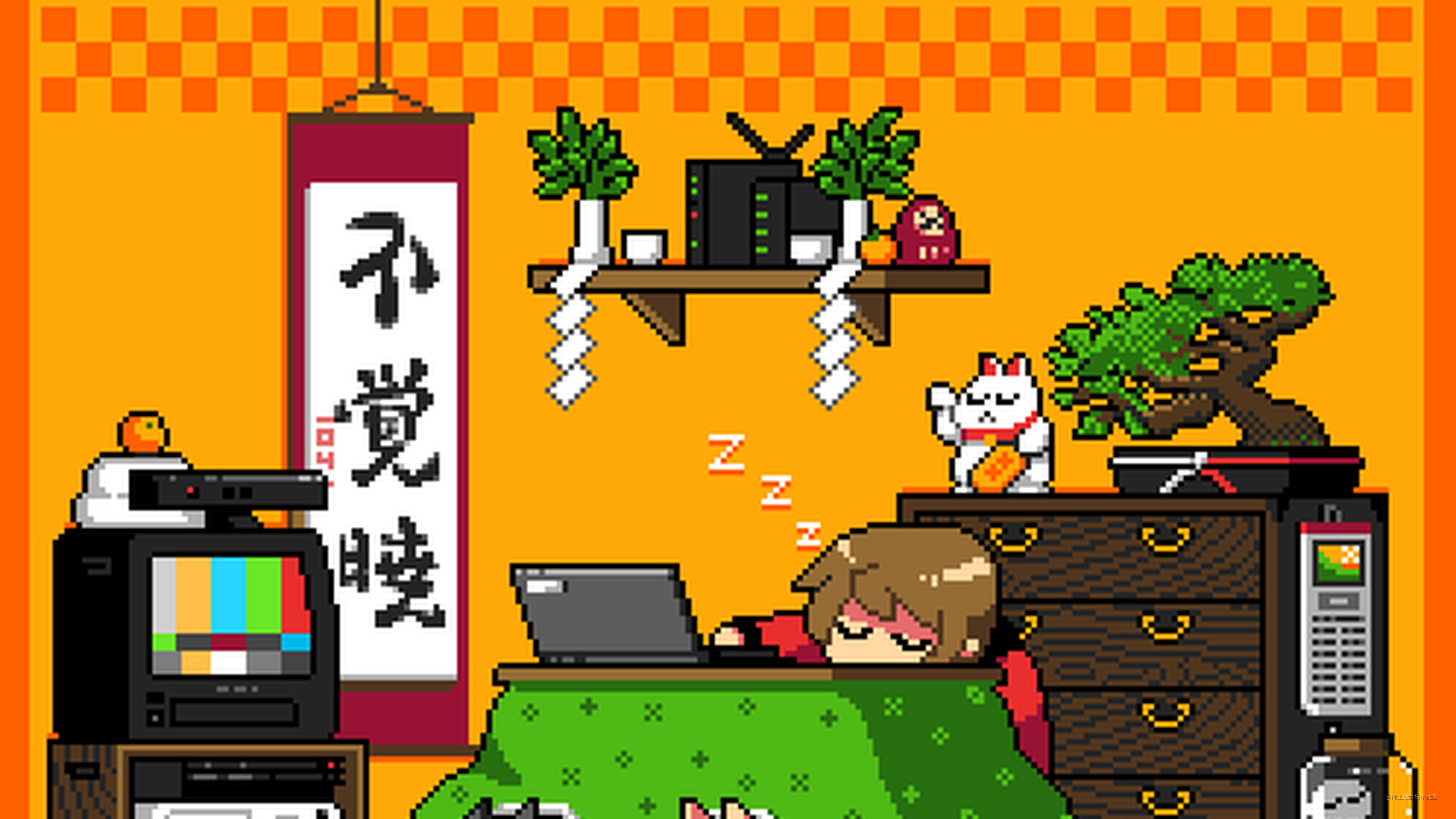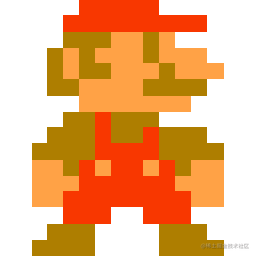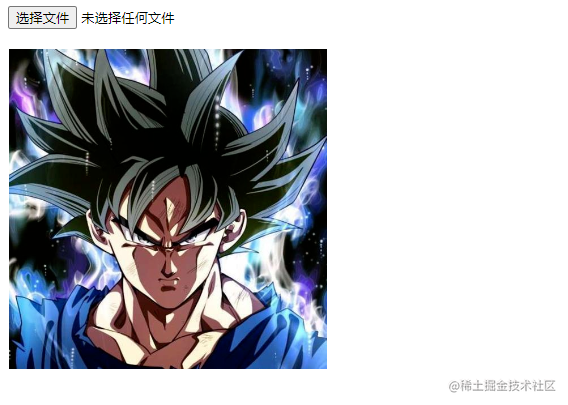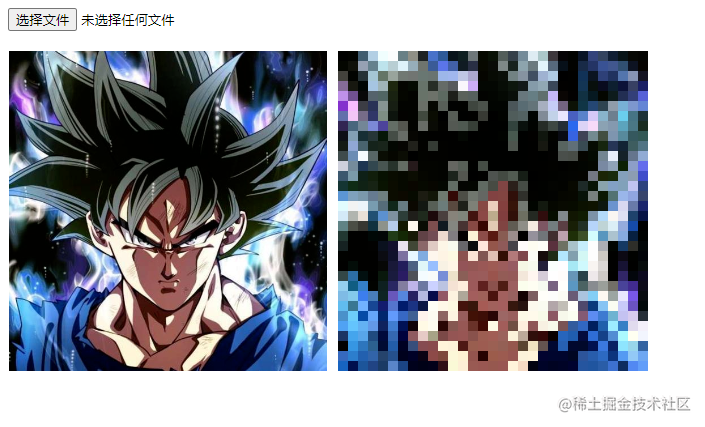# Canvas如何绘制像素风## 前言## 介绍所以我们第一就是上传一张图片，然后绘制出来。再解析每隔一定像素给图片取出色值和位置来。再将这些色值重新绘制到画布相应位置中，那么一张像素风就是这么简单实现了。

## 实现

### 1. 上传原图片

``````<input type="file" accept=".jpeg,.jpg,.png" />
<script>
</script>

``````function uploadImage(e) {
let file = e.target.files;
if (!file) return;
e.target.value = "";
document.querySelectorAll("canvas").forEach(node=>{node.remove();})
};
}

function createImage(obj) {
let img = new Image();
drawImage(img);
};
img.src = obj.result;
}

### 2.绘制原图片

``````function drawImage(img) {
let canvas = document.createElement("canvas");
let ctx = canvas.getContext("2d");
canvas.width = ctx.width;
canvas.height = ctx.heigh
let w = img.width,
h = img.height;
ctx.drawImage(img,0,0,w,h);
document.body.appendChild(canvas);
}``````let width = 32;
let height = 32;
let size = 10;

``````function drawImage(img) {
let canvas = document.createElement("canvas");
let ctx = canvas.getContext("2d");
canvas.width = ctx.width = width * size;
canvas.height = ctx.height = height * size;
let w = img.width,
h = img.height;
let v = w / h;
if (v > 1) {
w = width;
h = w / v;
} else {
h = height;
w = h * v;
}
ctx.drawImage(
img,
((width - w) / 2) * size,
((height - h) / 2) * size,
w * size,
h * size
);
document.body.appendChild(canvas);

let pxMap = createPxMap(ctx);
drawPXCanvas(pxMap)
}

### 3.绘制像素画

``````function createPxMap(ctx){
let pxMap = [];
for (let i = 0; i < width * size; i += size) {
for (let j = 0; j < height * size; j += size) {
let pixel = ctx.getImageData(i, j, 1, 1).data;
let color = `rgba(\${pixel},\${pixel},\${pixel},\${pixel/255})`;
pxMap.push({ x: i / size, y: j / size, color });
}
}
return pxMap;
}

``````function drawPXCanvas(pxMap) {
let canvas = document.createElement("canvas");
let ctx = canvas.getContext("2d");
canvas.width = ctx.width = width * size;
canvas.height = ctx.height = height * size;
pxMap.forEach((px) => {
const { color, x, y } = px;
ctx.fillStyle = color;
ctx.fillRect(x*size, y*size, size, size);
});
document.body.appendChild(canvas);
}## 拓展

像素孙悟空

像素小狐狸

2. 甚至我们也可以拓展webgl的，生成3d像素，或者其他风格也未尝不可。

3d皮卡丘

3. 我们可以将再深入分析像素化构成逐帧生成视频和动画。。。